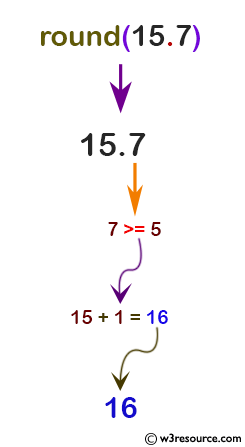# Python: round() function

## round() function

The round() function returns the rounded floating point value number, rounded to ndigits digits after the decimal point. If ndigits is omitted, it defaults to zero.

Note: For the built-in types supporting round(), values are rounded to the closest multiple of 10 to the power minus ndigits; if two multiples are equally close, rounding is done toward the even choice (so, for example, both round(0.5) and round(-0.5) are 0, and round(1.5) is 2). The return value is an integer if called with one argument, otherwise of the same type as number.

Version:

(Python 3.2.5)

Syntax:

```round(number[, ndigits])
```

Parameter:

Name Description Required /
Optional
number Number to be rounded. Required
ndigits The number of decimals to use. Default is 0 Optional

Return value:

Floating point value number.

Example-1: Python round() function

``````# for integers
print(round(5))

# for floating point
print(round(15.7))

# even choice
print(round(7.3))
``````

Output:

```5
16
7
```

Pictorial Presentation:Pictorial Presentation:Example-2: Python round() function

``````print(round(5.7754, 2))

# cannot be represented exactly as float
print(round(5.6758, 3))
``````

Output:

```5.78
5.676
```

Python Code Editor:

Previous: reversed()
Next: set()

Test your Python skills with w3resource's quiz

﻿

## Python: Tips of the Day

How do I check if a list is empty?

For example, if passed the following:

```a = []
if not a:
print("List is empty")
```

Ref: https://bit.ly/2A4JXx9